# How to Design an Efficient DC-DC Converter Using the DS1875 PWM Controller

### Abstract

The DS1875 features a pulse-width modulation (PWM) controller that can be used to control a DC-DC converter. The DC-DC converter can then be used to generate the high bias voltages necessary for avalanche photodiodes (APDs). This application note describes the operation of a boost converter that uses the DS1875. Discussion covers the selection of the inductor and switching frequency, and the selection of components that improve the efficiency of the converter. Test data are presented.

### Overview

The DS1875 features a pulse-width modulation (PWM) generator that can be used to control a DC-DC converter. In a typical application the PWM controller is used in a boost converter to generate biasing voltages for avalanche photodiodes (APDs). The PWM controller in the DS1875 can operate in either continuous or discontinuous conduction modes. To generate the high bias voltage needed for APDs, the DC-DC converter must operate in discontinuous mode. Figure 1 shows a simple boost converter using the DS1875 PWM controller.

This application note describes the operation of a boost converter with the DS1875. The article explains how to select both the inductor and switching frequency, and then the components that will improve the converter's efficiency.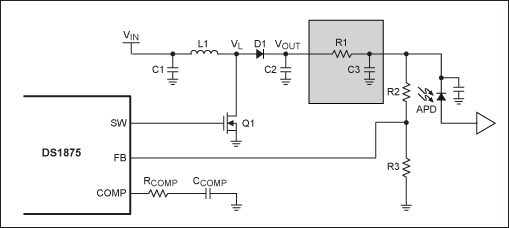### Boost Converter Operation

The DS1875 provides a PWM signal at the SW pin, which is used to drive the DC-DC converter. During each period of SW, a boost converter has a charge phase and a discharge phase. Figure 2 shows the inductor current and inductor voltage during both the charging and discharging phases.

The charging phase occurs when the transistor Q1 is turned on, placing the input voltage, VIN, across the inductor L1. Diode D1 prevents capacitor C2 from discharging to ground through Q1. Because the input voltage is DC, the inductor current rises linearly as seen in Figure 2. The equation for the inductor current is given by:The energy stored in the inductor during the charging phase is given by: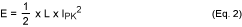IPK is the peak current that flows in the inductor. This current occurs at the end of the charging phase. Using Equation 1, this peak current can be calculated as:Where D and T are the duty cycle (%) and time period (s) of the switching frequency. Equation 3 can be substituted into Equation 2, giving the energy stored in the inductor during the charge phase:The discharge phase begins when Q1 is turned off. During the discharge phase, the energy stored in the inductor is transferred to the output. This energy is transferred because the inductor current continues flowing even after Q1 is switched off. The current now flows through diode D1 to output capacitor C2. For the inductor current to flow into the diode, the voltage at VL needs to be greater than the voltage at VOUT.

Figure 2 shows the large spike in the voltage at VL when the inductor current begins to flow through the diode. Now that there is a large negative voltage across the inductor, the slope of the current through the inductor reverses. Because of the large voltage across the inductor, the current in the inductor will quickly decrease to zero. Once all of the energy stored in the inductor has been injected into the output, inductor current decreases to zero. Because there is no more current to sustain the voltage at VL, this node drops back down to the input voltage, VIN. The inductor current during the discharge phase is given by:### Efficiency in a DC-DC Converter

There are many sources of loss in a DC-DC converter that reduce the efficiency of the system. These losses can be divided into two basic groups: efficiency losses caused by peak inductor current; and switching losses that occur each time that the circuit switches from charging to discharging phases. We will discuss each type of loss in turn.

There are three main sources of efficiency loss caused by inductor current. The two most significant sources are the drain-to-source resistance of the transistor when it is conducting, and the DC resistance of the inductor. Both of these actions add resistance in series with the inductor. These resistances consume input power and decrease the voltage across the inductor during the charging phase. When the inductor discharges through the diode, power loss proportional to the inductor current also occurs.

Switching losses occur during each cycle of the DC-DC converter. The most obvious switching losses are caused by parasitic capacitance in the circuit. Each time the inductor is discharged through the diode, the node at the anode of the diode needs to be charged to a voltage level greater than VOUT. This node also has the transistor's drain-to-source capacitance and the capacitance of the anode that both need to be charged before diode conduction can begin.

There are other sources of switching loss. Switching loss occurs at the beginning of each charging cycle when the transistor's gate capacitance needs to be charged before the transistor will turn on. Core loss in the inductor is another source of energy loss. As the switching frequency increases, so do the core losses in the inductor. The magnitude of these losses depends on inductor core material and size. Switching losses also occur during the reverse recovery time of the diode. During this time, charge that has been stored at the output is allowed to flow through the inductor.

### Minimizing Efficiency Losses

Proper component selection and PCB layout make a significant difference in the parasitic losses that occur. The operation of the system can also be managed by two different ways to improve efficiency. The first method is to reduce the inductor current, thus reducing the resistive losses of the inductor and transistor. The second method is to reduce the switching period of the system.

Inductor current can be reduced in a DC-DC converter by increasing the size of the inductor and the duty cycle at which the system operates. The duty cycle and inductor size can be changed as long as the same amount of energy is stored by the inductor and then injected to the output each time period. Figure 3 shows three inductors (1, 2, and 4 Henrys, respectively), each with 1V applied to them, charging until they store 2 Joules of energy. The 4H inductor takes twice as long, or twice the duty cycle, as the 1H inductor to charge to the same stored energy level of 2 Joules.

Figure 4 shows the current flowing in these three inductors as they are charging. It can be seen that the 1H inductor requires 2A of current, but the 4H inductor only requires 1A of current. This illustrates how choosing a larger inductor and increasing the duty cycle of a DC-DC converter reduces inductor current.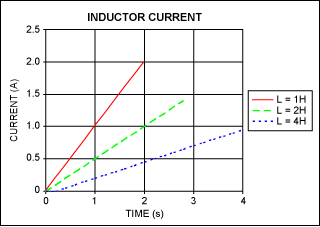Switching losses in a converter occur each time that the circuit switches. These losses are independent of the inductor current, and are directly proportional to the switching period. Therefore, it is advantageous to use the longest switching period that allows for proper system operation. However, a longer switching period requires a larger inductor value. Increasing the inductor value will increase the DC resistance of the inductor and may necessitate using an inductor that is also physically larger.

Figure 5 shows the efficiency versus load current for a DC-DC converter using the DS1875's PWM controller. This graph shows that increasing the inductance, which increases the duty cycle, increased the efficiency. It also shows that a longer time period resulted in a higher efficiency because the switching losses were reduced. This example converter uses a BSSS123 n-channel FET and a 1N4148 diode. Table 1 shows parameters for the two inductors used.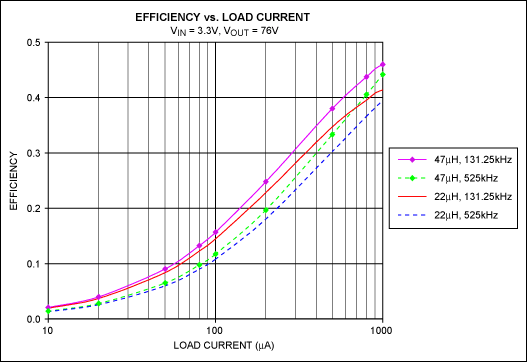Inductor Value (µH Current Rating (mA) DC Resistance (Ω) Package Size (mils) 47 390 0.67 1210 22 175 0.44 1007

### Selection of Inductor, Duty Cycle, and Time Period

The first step in setting up a boost converter is to determine the desired output voltage and the maximum current needed by the load. The available energy from the boost converter needs to be greater than the required output energy and all of the combined losses in the circuit. Converter efficiency is represented by η. An efficiency of 0.4 to 0.75 is a suitable starting point when calculating circuit components and settings. The total energy required each time period is:Setting the input energy (Equation 4) and the output energy (Equation 6) equal to each other and then dividing both sides by T gives an equation for the energy equality in the system:From Equation 7, the required inductor value can be calculated: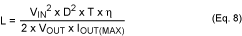It is desirable to operate the DC-DC converter as close to the maximum duty cycle as possible. The DS1875 offers a maximum duty cycle of 90%. A properly setup DC-DC converter system using the DS1875 should have a duty cycle of between 80 and 85%. This will reduce the inductor current as much as possible, but still leave extra duty-cycle margin if the efficiency is less than expected.

The time period should be as long as possible to reduce switching losses. The DS1875 offers four different switching frequencies to choose from: 131.25kHz, 262.5kHz, 525kHz, and 1050kHz. The trade-offs between a longer switching period and a larger inductance value must be considered when choosing an operating frequency and inductor for a DC-DC converter.

### Example Calculations for Typical DC-DC Converters

Following is a calculation using system requirements and initial assumptions to determine the inductor needed for a particular application.

 Requirements Initial Assumptions VIN = 3.3V D = 80% VOUT = 76V T = 1/262.5kHz IOUT = 5mA η = 0.5

Using the formula in Equation 8, an inductor value of 17.5µH is calculated. A standard value of 15µH is chosen. Table 2 shows other common DC-DC converter configurations using the DS1875 as the PWM controller. The table shows the calculated inductance and the switching frequency that were selected. This table is calculated using an efficiency of 50% and a target duty cycle of 80%.

 VIN (V) VOUT (V) IOUT (mA) Switching Freq (kHz) Inductor (µH) 3.3 76 5 262.5 15 3.3 38 5 525 15 12 76 5 1050 56

### Diode Selection

Four parameters must be considered when selecting a diode for a DC-DC converter application. First, the reverse breakdown voltage of the diode must be greater than the voltage at the output of the converter. Second, the diode must be able to operate with the forward current that will be pushed through the diode by the inductor. In a DC-DC converter, this current (IPK) can be several hundred milliamps. Third, to minimize power loss when the diode is conducting, the forward voltage should be as small as possible. Some applications may even use Schottky diodes because they have a much lower forward voltage. Finally, choosing a diode with a short reverse-recovery time will limit the output charge lost back to the input when the diode switches from the conducting to the nonconducting stage.

### Inductor Selection

When selecting the inductor for a DC-DC converter, three parameters should be considered. First, the most critical parameter is the saturation current of the inductor. If the saturation current of the inductor is less than the converter's required peak current, then the converter will not be able to supply the necessary output power. Second, the designer must consider the DC resistance of the inductor. Finally, the physical size of the inductor should be considered. In order to lower the DC resistance, an inductor in a larger package could be considered if the physical size of the inductor is not prohibitive.

Final selection of the inductor will depend upon the key requirements of the DC-DC converter.

### Transistor Selection

The transistor selected must be able to withstand the drain-to-source voltage that occurs when the inductor is discharging through the diode. The rated drain current of the transistor needs to be greater than the peak inductor current. To minimize switching losses, an optimal transistor will also have low gate-to-source and drain-to-source capacitances.

### Ripple Filter Selection

A filter comprised of R1 and C3 can be used for ripple rejection. The DS1875 data sheet describes how to select these components. The selection of R1 can have a significant effect on system efficiency. Depending on the R1 value chosen, a large amount of power can be consumed by R1 when an APD is sinking a high current.

### PCB Layout

Proper PCB layout can decrease the capacitance at the switching nodes of a DC-DC converter. Reducing the capacitance decreases the switching losses, thus improving efficiency. Proper layout can also reduce the switching noise that is generated by a DC-DC converter. The following application notes discuss the layout of a DC-DC converter in more detail.

Application note 735, "Layout Considerations for Non-Isolated DC-DC Converters."

Application note 3645, "Correct Board Layout Lowers EMI of Switchmode Converters."

Application note 716, "Proper Layout and Component Selection Controls EMI."